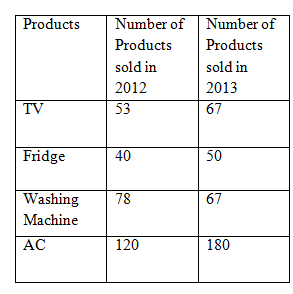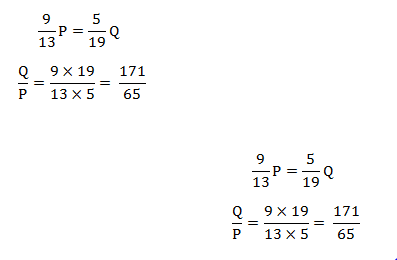# SSC CGL 2018 Practice Test Papers | Quantitative Aptitude (Day-52)

Dear Aspirants, Here we have given the Important SSC CGL Exam 2018 Practice Test Papers. Candidates those who are preparing for SSC CGL 2018 can practice these questions to get more confidence to Crack SSC CGL 2018 Examination.

[WpProQuiz 3597]

Click “Start Quiz” to attend these Questions and view Explanation

1) If (x – 5)2 + (y -1)2 + (z +3)2 = 0, then what is the value of 2x + y + z – 2?

a) 4

b) 6

c) 2

d) 8

2) What is the value of tan 32° sin 26° cos 45° sin 64° tan 58°?

a) 1/2

b) 1/√2

c) √3

d) None of the above

3) If 2NN4 is divisible by 8, then which of the following can be the value of N?

a) 2

b) 6

c) Both a and b

d) None of these

4) A solid sphere  is cut into two equal halves. What will be the increase (in cm2 ) in the total surface area if the diameter of the sphere is 18.2 cm?

a) 296.78

b) 341.45

c) 260.26

d) 94.94

5) After a discount of 4.17% an article is sold for Rs. 10327. What is the cost price if the seller got 9.09% profit without giving any discount ?

a) 9878

b) 12300

c) 8467

d) 10200

6) If 9/13 P= 5/19 Q What is the ratio of Q: P?

a) 123: 67

b) 171:65

c) 167: 65

d) None of these

7) The average of 15 results is 120. If the average of first 8 results is 130 and that of the last 8 results is 142, then what will be the value of 25% of 8th result?

a) 100

b) 376

c) 94

d) 125

Direction : (8-10)

The sale of four different products in two different years of a electronics firm is given below in the following table chart.   Study the chart and answer the following questions.8) The Seller gets a profit of 25% on TV and the ratio of Selling Price of TV in 2012 and 2013 is 5:7. If the Cost price of TV in 2012 is 20000 find the selling Price of TV in 2013

a) 35000

b) 20000

c) 25000

d) 30000

9) What is the sum of TV and Fridge sold in 2012 and Washing Machine and Fridge sold in the year 2013?

a) 253

b) 421

c) 323

d) 289

10) What is the ratio of 75% of AC sold in 2012 to 180% of Fridge sold in 2013?

a) 1:1

b) 2:1

c) 1:2

d) 1:3

(x – 5)2 + (y -1)2 + (z +3)2 = 0

If ( x-a)n=0 , it is clear that x=-a

(x – 5)2 + (y -1)2 + (z +3)2 = 0

X=5,y=1, z=-3

2x + y + z – 2=2(5)+1+(-3)-2=6

tan(90-x)=cot x

Sin(90-x)=cos x

Sin x. cosx =1

Tanx .cot x=1

tan 32° sin 26° cos 45° sin 64° tan 58°

=tan 32° sin 26° cos 45° sin (90-26°) tan (90-32°)

= tan 32°tan (90-32°)sin 26°sin (90-26°)cos 45°

= tan 32° cot 32°sin 26°cos 26ocos 45°

=1.1. cos 45°

= cos 45°

=1/√2

Divisibility rule of 8 is last three digits of the number should be divisible by 8.

If n=2 , the number is 2224. It is divisible by 8

If n=6 , the number is 2664. It is divisible by 8

Diameter of Sphere= 18.2

T.S.A of Sphere= 4πr2

T.S.A of sphere =4×22/7×9.1×9.1=1041.04

T.S.A of Hemisphere= 3πr2

T.S.A of 2 hemisphere=2(3×22/7×9.1×9.1)=780.78

1041.04-780.78=260.26 cm2

Hence,the correct answer is 260.26 cm2

Let the marked Price and cost price be x and y respectively.

4.17%=1/24

9.09%=1/11

Selling Price = Marked Price- Discount= x-x/24=23x/24

10327——–23

x————–24

x=24×10327/23=10776

Marked Price= 10776

Without any discount Selling Price = Marked Price=10776

Cost Price= Marked Price –Profit

y+(y/11)= 10776

12y/11=10776

y=9878Average of 15 results= 120

Sum of 15 results=120×15=1800

Average of first 8 results= 130

Sum of first 8 results=130×8=1040

Average of last 8 results= 142

Sum of last 8 results=142×8=1136

Eighth result =1040+1136-1800=376

25% of 8th result= 1/4×376=94

Direction (8-10) :

Cost Price of TV in 2012

Selling Price of Tv in 2012= 125/100 x20000= 25000

Selling Price of Tv in 2012: Selling Price of Tv in 2013 =5: 7

5——25000

7——-?

?= 7×25000/5

?=35000

Selling Price of Tv in 2013= 35000

TV and Fridge sold in 2012= 53+40= 93

Washing Machine and Fridge sold in the year 2013=50+180=230

Sum= 230+ 93=323

AC sold in 2012=120

75% of AC sold in 2012=75/100×120=90

Fridge sold in 2013=50

180% of Fridge sold in 2013= 180/100× 50= 90

Ratio= 90: 90

=1:1